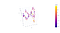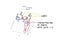# Hands on with COVID-19 drug discovery data set

## STEP 1. Building a point cloud

`features = clean.loc[:, :'pIC50']n_neighbors=10min_dist=0.5umap_2d = umap.UMAP(n_neighbors=n_neighbors                    n_components=2,                    min_dist=min_dist,                    init='random',                    random_state=0) umap_3d = umap.UMAP(n_neighbors=n_neighbors,                    n_components=3,                    min_dist=min_dist,                    init='random',                    random_state=0)proj_2d = umap_2d.fit_transform(clean.drop(columns='pIC50'))proj_3d = umap_3d.fit_transform(clean.drop(columns='pIC50'))fig_2d = px.scatter( proj_2d, x=0, y=1,                   color=clean['pIC50'],                    labels={'color': 'pIC50'})fig_3d = px.scatter_3d(proj_3d, x=0, y=1, z=2,                      color=clean['pIC50'],                       labels={'color': 'pIC50'})fig_2d.update_layout(title='UMAP projection 2D and 3D')fig_3d.update_traces(marker_size=5)fig_2d.update_layout({'plot_bgcolor': 'aliceblue' , 'paper_bgcolor': 'white',}, template='plotly_white')fig_3d.update_layout({'plot_bgcolor': 'aliceblue' , 'paper_bgcolor': 'white',}, template='plotly_white')fig_2d.show()fig_3d.show()`Figure 8. Dataset weighted graph with UMAP. Color scale corresponds to pIC50 valuesFigure 9. Dataset weighted graph with UMAP. Color scale corresponds to binarized pIC50 values

## Mapper

`#build a pipeline for mapper algorithmmake_mapper_pipeline(filter_func,                     cover,                     clusterer)`
`""" 1. Define filter function – can be any scikit-learn transformer.It is returning a selection of columns of the data """filter_func = Eccentricity(metric= 'euclidean') #Eccentricities of points in a point cloud or abstract metric space.""" 2. Define cover """cover = CubicalCover(n_intervals=30, overlap_frac=0.3)""" 3. Choose clustering algorithm – default is DBSCAN """clusterer = DBSCAN(eps=8, min_samples=3, metric='euclidean')""" 4. Initialise pipeline """pipe_mapper = make_mapper_pipeline( filter_func=filter_func, cover=cover, clusterer=clusterer, verbose=False, n_jobs=-1)`
`data = clean.drop(columns='pIC50')#Check the cluster performancedb = clusterer.fit(data)labels = db.labels_n_clusters_ = len(set(labels)) - (1 if -1 in labels else 0)n_noise_ = list(labels).count(-1)print('Estimated number of clusters: %d' % n_clusters_)print('Estimated number of noise points: %d' % n_noise_)"""The best value of Silhouette score is 1, and the worst value is -1. Values near 0 indicate overlapping clusters. Negative values generally indicate that a sample has been assigned to the wrong cluster, as a different cluster is more similar."""print("Silhouette Coefficient: %0.3f" % metrics.silhouette_score(proj_3d, labels))`
`Estimated number of clusters: 1Estimated number of noise points: 88Silhouette Coefficient: 0.003`
`plotly_params = {"node_trace": {"marker_colorscale": "RdBu"}}fig = plot_static_mapper_graph(pipe_mapper, data, layout='fruchterman_reingold', color_by_columns_dropdown=True, color_variable =clean['pIC50'], node_scale =20, plotly_params=plotly_params)fig.show(config={'scrollZoom': True})`